# Vehicle Steering Gain at Different Speeds

This example shows how to use the vehicle dynamics slowly increasing steering reference application to analyze the impact of the steering angle and speed on vehicle handling. Specifically, you can calculate the steering gain when you run the maneuver with different speed set points. Based on the constant speed, variable steer test defined in SAE J266, the slowly increasing steering maneuver helps characterize the lateral dynamics of the vehicle. In the test, the driver:

• Accelerates until vehicle hits a target velocity.

• Maintains a target velocity.

• Linearly increases the steering wheel angle from 0 degrees to a maximum angle.

• Maintains the steering wheel angle for a specified time.

• Linearly decreases the steering wheel angle from maximum angle to 0 degrees.

```helpersetupsis; ```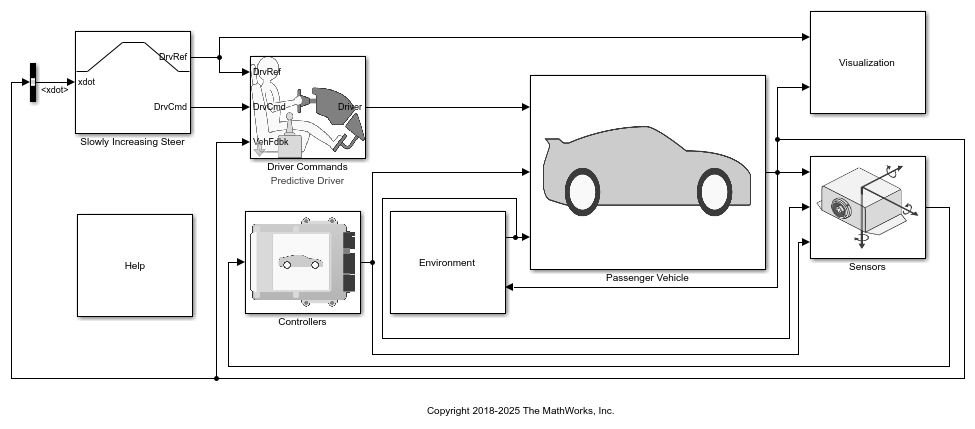### Run a Slowly Increasing Steering Maneuver

1. Open the Swept Sine Reference Generator block. By default, the maneuver is set with these parameters:

• Longitudinal speed setpoint — 50 mph

• Handwheel rate — 13.5 deg

• Maximum handwheel angle — 270 deg

2. In the Visualization subsystem, open the 3D Engine block. By default, the 3D Engine parameter is set to Disabled. For the 3D visualization engine platform requirements and hardware recommendations, see the Unreal Engine Simulation Environment Requirements and Limitations.

3. Run the maneuver with the default settings. As the simulation runs, view the vehicle information.

```mdl = 'ISReferenceApplication'; sim(mdl); ```
```### Starting serial model reference simulation build ### Successfully updated the model reference simulation target for: Driveline ### Successfully updated the model reference simulation target for: PassVeh14DOF ### Successfully updated the model reference simulation target for: SiMappedEngineV Build Summary Simulation targets built: Model Action Rebuild Reason ========================================================================================== Driveline Code generated and compiled Driveline_msf.mexw64 does not exist. PassVeh14DOF Code generated and compiled PassVeh14DOF_msf.mexw64 does not exist. SiMappedEngineV Code generated and compiled SiMappedEngineV_msf.mexw64 does not exist. 3 of 3 models built (0 models already up to date) Build duration: 0h 5m 28.185s ```• In the Vehicle Position window, view the vehicle longitudinal distance as a function or the lateral distance. The yellow line displays the yaw rate. The blue line shows the steering angle.

• In the Visualization subsystem, open the Yaw Rate and Steer Scope block to display the yaw rate and steering angle versus time.### Sweep Speed Set Points

Run the slowly increasing steering angle reference application with three different speed set points.

1. In the slowly increasing steering reference application model ISReferenceApplication, open the Slowly Increasing Steer block. The Longitudinal speed set point, xdot_r block parameter sets the vehicle speed. By default, the speed is 50 mph.

2. Enable signal logging for the velocity, lane, and ISO signals. You can use the Simulink® editor or, alternatively, these MATLAB® commands. Save the model.

• Enable signal logging for the Slowly Increasing Steer Ref signal outport.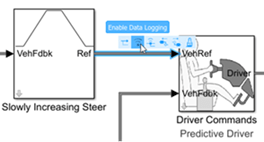```mdl = 'ISReferenceApplication'; ph=get_param('ISReferenceApplication/Slowly Increasing Steer','PortHandles'); set_param(ph.Outport(1),'DataLogging','on'); ```
• Enable signal logging for the Passenger Vehicle block outport signal.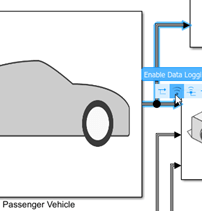```ph=get_param('ISReferenceApplication/Passenger Vehicle','PortHandles'); set_param(ph.Outport(1),'DataLogging','on'); ```
• In the Visualization subsystem, enable signal logging for the ISO block.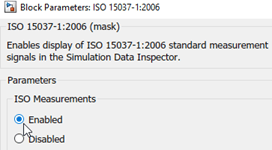```set_param([mdl '/Visualization/ISO 15037-1:2006'],'Measurement','Enable'); ```

3. Set up a speed set point vector, `xdot_r`, that you want to investigate. For example, at the command line, type:

```vmax = [45, 50, 55]; numExperiments = length(vmax); ```

4. Create an array of simulation inputs that set the Swept Sine Reference Generator block parameter Steering amplitude, theta_hw equal to `amp`.

```for idx = numExperiments:-1:1 in(idx) = Simulink.SimulationInput(mdl); in(idx) = in(idx).setBlockParameter([mdl '/Slowly Increasing Steer'], ... 'xdot_r', num2str(vmax(idx))); end ```

5. Save the model and run the simulations. If available, use parallel computing.

```save_system(mdl) tic; simout = parsim(in,'ShowSimulationManager','on'); toc; ```
```[23-Jun-2021 15:45:12] Checking for availability of parallel pool... [23-Jun-2021 15:45:12] Starting Simulink on parallel workers... [23-Jun-2021 15:45:14] Loading project on parallel workers... [23-Jun-2021 15:45:14] Configuring simulation cache folder on parallel workers... [23-Jun-2021 15:45:14] Loading model on parallel workers... [23-Jun-2021 15:45:27] Running simulations... [23-Jun-2021 15:46:21] Completed 1 of 3 simulation runs [23-Jun-2021 15:46:21] Completed 2 of 3 simulation runs [23-Jun-2021 15:46:22] Completed 3 of 3 simulation runs [23-Jun-2021 15:46:22] Cleaning up parallel workers... Elapsed time is 81.594518 seconds. ```

6. After the simulations complete, close the Simulation Data Inspector windows.

### Use Simulation Data Inspector to Analyze Results

Use the Simulation Data Inspector to examine the results. You can use the UI or, alternatively, command-line functions.

1. Open the Simulation Data Inspector. On the Simulink Toolstrip, on the Simulation tab, under Review Results, click Data Inspector.

• In the Simulation Data Inspector, select Import.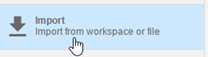• In the Import dialog box, clear `logsout`. Select `simout(1)`, `simout(2)`, and `simout(3)`. Select Import.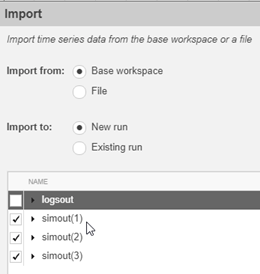• Use the Simulation Data Inspector to examine the results.

2. Alternatively, use these MATLAB commands to plot the longitudinal velocity, steering wheel angle, lateral acceleration, longitudinal position, and lateral position.

```for idx = 1:numExperiments % Create sdi run object simoutRun(idx)=Simulink.sdi.Run.create; simoutRun(idx).Name=['Velocity = ', num2str(vmax(idx))]; add(simoutRun(idx),'vars',simout(idx)); end sigcolor=[0 1 0;0 0 1;1 0 1]; for idx = 1:numExperiments % Extract the lateral acceleration, position, and steering xsignal(idx)=getSignalByIndex(simoutRun(idx),22); xsignal(idx).LineColor =sigcolor((idx),:); ysignal(idx)=getSignalByIndex(simoutRun(idx),23); ysignal(idx).LineColor =sigcolor((idx),:); msignal(idx)=getSignalByIndex(simoutRun(idx),256); msignal(idx).LineColor =sigcolor((idx),:); ssignal(idx)=getSignalByIndex(simoutRun(idx),255); ssignal(idx).LineColor =sigcolor((idx),:); asignal(idx)=getSignalByIndex(simoutRun(idx),251); asignal(idx).LineColor =sigcolor((idx),:); end Simulink.sdi.view Simulink.sdi.setSubPlotLayout(5,1); for idx = 1:numExperiments % Plot the lateral position, steering angle, and lateral acceleration plotOnSubPlot(msignal(idx),1,1,true); plotOnSubPlot(ssignal(idx),2,1,true); plotOnSubPlot(asignal(idx),3,1,true); plotOnSubPlot(xsignal(idx),4,1,true); plotOnSubPlot(ysignal(idx),5,1,true); end ```

The results are similar to these plots, which indicate that the greatest lateral acceleration occurs when the vehicle velocity is 45 mph.### Further Analysis

To explore the results further, use these commands to extract the lateral acceleration, steering angle, and vehicle trajectory from the `simout` object.

1. Extract the lateral acceleration and steering angle. Plot the data. The results are similar to this plot.

```figure for idx = 1:numExperiments % Extract Data log = get(simout(idx),'logsout'); sa=log.get('Steering-wheel angle').Values; ay=log.get('Lateral acceleration').Values; firstorderfit = polyfit(sa.Data,ay.Data,1); gain(idx)=firstorderfit(1); legend_labels{idx} = [num2str(vmax(idx)), ' mph: Gain = ', ... num2str(gain(idx)), ' m/(deg s^2)']; % Plot steering angle vs. lateral acceleration plot(sa.Data,ay.Data) hold on end % Add labels to the plots legend(legend_labels, 'Location', 'best'); title('Lateral Acceleration') xlabel('Steering Angle [deg]') ylabel('Acceleration [m/s^2]') grid on ```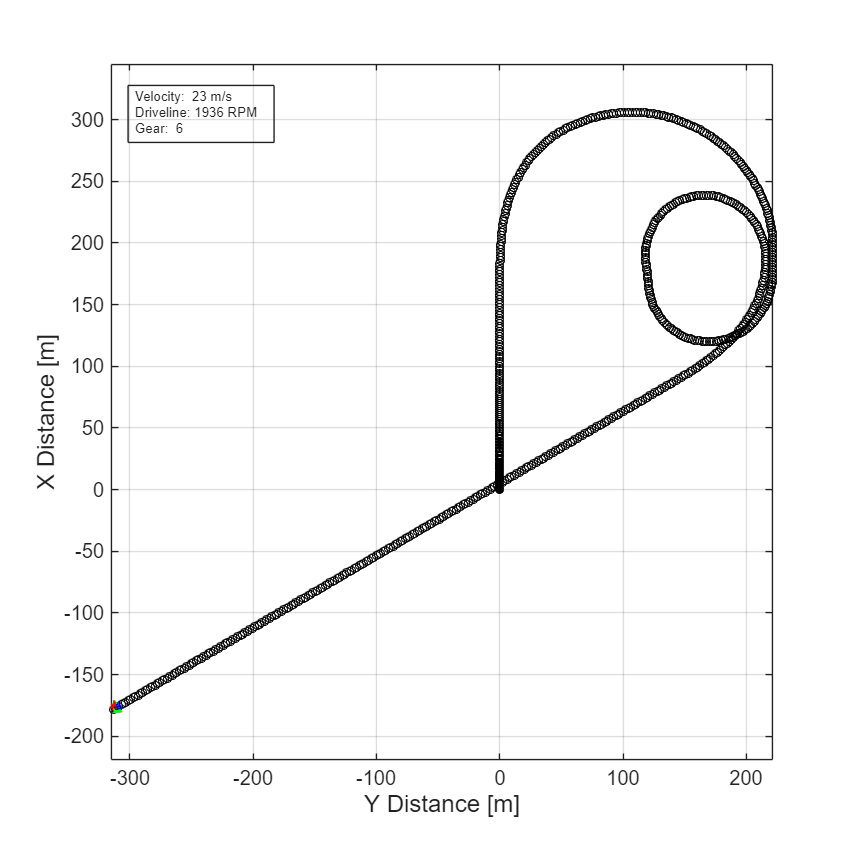2. Extract the vehicle path. Plot the data. The results are similar to this plot.

```figure for idx = 1:numExperiments % Extract Data log = get(simout(idx),'logsout'); x = log{1}.Values.Body.InertFrm.Cg.Disp.X.Data; y = log{1}.Values.Body.InertFrm.Cg.Disp.Y.Data; legend_labels{idx} = [num2str(vmax(idx)), ' mph']; % Plot vehicle location axis('equal') plot(y,x) hold on end % Add labels to the plots legend(legend_labels, 'Location', 'best'); title('Vehicle Path') xlabel('Y Position [m]') ylabel('X Position [m]') grid on ```SAE J266. Steady-State Directional Control Test Procedures For Passenger Cars and Light Trucks. Warrendale, PA: SAE International, 1996.